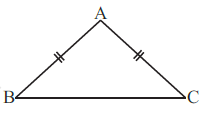Q

# ABC is an isosceles triangle with AB is equal to AC (fig 7.17). Take a trace-copy of Delta ABC and also name it as Delta ABC

1.  ABC is an isosceles triangle with  (Fig 7.17). Take a trace-copy of  and also name it as
(i) State the three pairs of equal parts in .
(ii) Is ? Why or why not?
(iii) Is  ? Why or why not?Views

Here, in .i)the three pairs of equal parts in  are

AB = AC

BC = CB

AC = AB

ii)

Hence By SSS Congruency rule, they both are congruent.

iii) Yes,  because  are congruent and by equating the corresponding parts of the triangles we get,

.

Exams
Articles
Questions